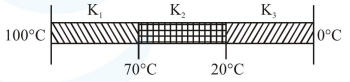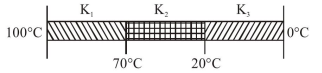# Three rods of identical cross-section and lengths

Question:

Three rods of identical cross-section and lengths are made of three different materials of thermal conductivity $\mathrm{K}_{1}, \mathrm{~K}_{2}$, and $\mathrm{K}_{3}$, respectively. They are joined together at their ends to make a long rod (see figure). One end of the long rod is maintained at $100^{\circ} \mathrm{C}$ and the other at $0^{\circ} \mathrm{C}$ (see figure). If the joints of the rod are at $70^{\circ} \mathrm{C}$ and $20^{\circ} \mathrm{C}$ in steady state and there is no loss of energy from the surface of the rod, the correct relationship between $\mathrm{K}_{1}, \mathrm{~K}_{2}$ and $\mathrm{K}_{3}$ is :1. $\mathrm{K}_{1}: \mathrm{K}_{3}=2: 3 ; \mathrm{K}_{2}: \mathrm{K}_{3}=2: 5$

2. $\mathrm{K}_{1}<\mathrm{K}_{2}<\mathrm{K}_{3}$

3. $\mathrm{K}_{1}: \mathrm{K}_{2}=5: 2 ; \mathrm{K}_{1}: \mathrm{K}_{3}=3: 5$

4. $\mathrm{K}_{1}>\mathrm{K}_{2}>\mathrm{K}_{3}$

Correct Option: 1

Solution:Rods are identical have same length $(\ell)$ and area of cross-section (A)

Combination are in series, so heat current is same for all Rods

$\left(\frac{\Delta \mathrm{Q}}{\Delta \mathrm{t}}\right)_{\mathrm{AB}}=\left(\frac{\Delta \mathrm{Q}}{\Delta \mathrm{t}}\right)_{\mathrm{BC}}=\left(\frac{\Delta \mathrm{Q}}{\Delta \mathrm{t}}\right)_{\mathrm{CD}}=$ Heat current

$\frac{(100-70) \mathrm{K}_{1} \mathrm{~A}}{\ell}=\frac{(70-20) \mathrm{K}_{2} \mathrm{~A}}{\ell}=\frac{(20-0) \mathrm{K}_{3} \mathrm{~A}}{\ell}$

$30 \mathrm{~K}_{1}=50 \mathrm{~K}_{2}=20 \mathrm{~K}_{3}$

$3 \mathrm{~K}_{1}=2 \mathrm{~K}_{3}$

$\frac{\mathrm{K}_{1}}{\mathrm{~K}_{3}}=\frac{2}{3}=2: 3$

$5 \mathrm{~K}_{2}=2 \mathrm{~K}_{3}$

$\frac{\mathrm{K}_{2}}{\mathrm{~K}_{3}}=\frac{2}{5}=2: 5$